# 15 Multiple Choice Calculus Equation Questions & Solutions

1.

Evaluate the integral: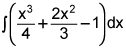(4 points)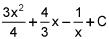x4 + 2x3 – x + C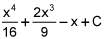2.

Evaluate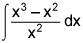. (4 points)

 x – 1 + C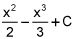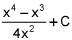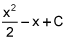3.

Evaluate the integral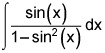. (4 points)

 2ln|1 – sin2(x)| + C -csc(x) + C sec(x) + C None of these

4.

If f(x) and g(x) are continuous on [a, b], which one of the following statements is true? (4 points)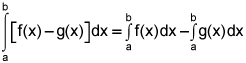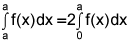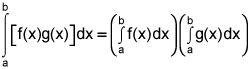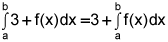5.

Evaluate the integral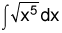. (4 points)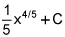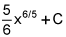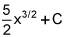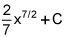1.

Evaluate the integral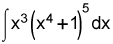. (4 points)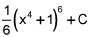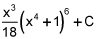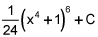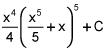2.

Evaluate the integral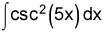. (4 points)

 -2csc(5x)cot(5x) + C -10csc(5x)cot(5x) + C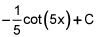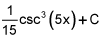3.

Which of the following integrals cannot be evaluated using a simple substitution? (4 points)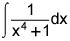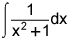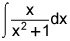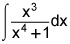4.

Evaluate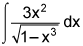. (4 points)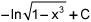-ln|1 – x3| + C sin-1(x3) + C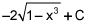5.

Evaluate the integral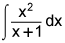. (4 points)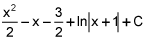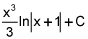x – 1 + ln|x| + C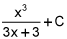1.

Which of the following definite integrals could be used to calculate the total area bounded by the graph of y = 1 – x2 and the x-axis? (4 points)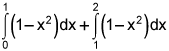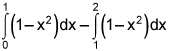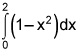2.

Suppose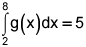, and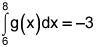, find the value of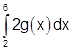. (4 points)

 8 12 16 4

3.

Evaluate the integral . (4 points)

 1 0 2 -1

4.

Use your graphing calculator to evaluate to three decimal places the value of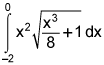. (4 points)

 0.25 2.667 1.778 -3.251

5.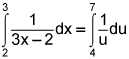(4 points)

 True False